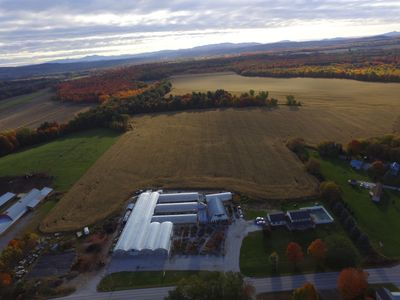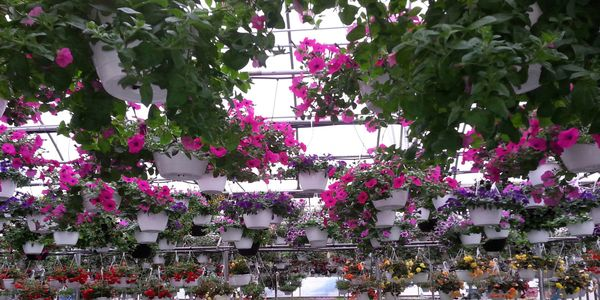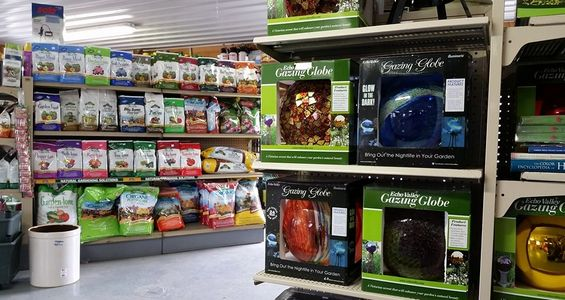# Celebrating 25 years of Growing! 1994-2019

H & B H & B H & B H & B H & B H & B H & B H & B H & B H & B H & B H & B H & B H & B H & B H & B H & B H & B H & B H & B H & B H & B H & B H & B H & B H & B H & B H & B H & B H & B H & B H & B H & B H & B H & B H & B H & B H & B H & B H & B H & B H & B H & B H & B H & B H & B H & B H & B H & B H & B H & B H & B H & B H & B H & B H & B H & B H & B H & B H & B H & B H & B H & B H & B H & B H & B H & B H & B H & B H & B H & B H & B H & B H & B H & B H & B H & B H & B H & B H & B H & B H & B H & B H & B H & B H & B H & B H & B H & B H & B H & B H & B H & B H & B H & B H & B H & B H & B H & B H & B H & B H & B H & B H & B H & B H & B H & B H & B H & B H & B H & B H & B H & B H & B H & B H & B H & B H & B H & B H & B H & B H & B H & B H & B H & B H & B H & B H & B H & B H & B H & B H & B H & B H & B H & B H & B H & B H & B H & B H & B H & B H & B H & B H & B H & B H & B H & B H & B H & B H & B H & B H & B H & B H & B H & B H & B H & B H & B H & B H & B H & B H & B H & B H & B H & B H & B H & B H & B H & B H & B H & B H & B H & B H & B H & B H & B H & B H & B H & B H & B H & B H & B H & B H & B H & B H & B H & B H & B H & B H & B H & B H & B H & B H & B H & B H & B H & B H & B H & B H & B H & B H & B H & B H & B H & B H & B H & B H & B H & B H & B H & B H & B H & B H & B H & B H & B H & B H & B H & B H & B H & B H & B H & B H & B H & B H & B H & B H & B H & B H & B H & B H & B H & B H & B H & B H & B H & B H & B H & B H & B H & B H & B H & B H & B H & B H & B H & B H & B H & B H & B H & B H & B H & B H & B H & B H & B H & B H & B H & B H & B H & B H & B H & B H & B H & B H & B H & B

# Your Locally owned garden center for 25 years!

H & B Greenhouse & Nursery

Highgate Center, VT 05459

(802) 868-3604

H & B H & B H & B H & B H & B H & B H & B H & B H & B H & B H & B H & B H & B H & B H & B H & B H & B H & B H & B H & B H & B H & B H & B H & B H & B H & B H & B H & B H & B H & B H & B H & B H & B H & B H & B H & B H & B H & B H & B H & B H & B H & B H & B H & B H & B H & B H & B H & B H & B H & B H & B H & B H & B H & B H & B H & B H & B H & B H & B H & B H & B H & B H & B H & B H & B H & B H & B H & B H & B H & B H & B H & B H & B H & B H & B H & B H & B H & B H & B H & B H & B H & B H & B H & B H & B H & B H & B H & B H & B H & B H & B H & B H & B H & B H & B H & B H & B H & B H & B H & B H & B H & B H & B H & B H & B H & B H & B H & B H & B H & B H & B H & B H & B H & B H & B H & B H & B H & B H & B H & B H & B H & B H & B H & B H & B H & B H & B H & B H & B H & B H & B H & B H & B H & B H & B H & B H & B H & B H & B H & B H & B H & B H & B H & B H & B H & B H & B H & B H & B H & B H & B H & B H & B H & B H & B H & B H & B H & B H & B H & B H & B H & B H & B H & B H & B H & B H & B H & B H & B H & B H & B H & B H & B H & B H & B H & B H & B H & B H & B H & B H & B H & B H & B H & B H & B H & B H & B H & B H & B H & B H & B H & B H & B H & B H & B H & B H & B H & B H & B H & B H & B H & B H & B H & B H & B H & B H & B H & B H & B H & B H & B H & B H & B H & B H & B H & B H & B H & B H & B H & B H & B H & B H & B H & B H & B H & B H & B H & B H & B H & B H & B H & B H & B H & B H & B H & B H & B H & B H & B H & B H & B H & B H & B H & B H & B H & B H & B H & B H & B H & B H & B H & B H & B H & B H & B H & B H & B H & B H & B H & B H & B H & B H & B H & B H & B H & B H & B

# Your Locally owned garden center for 25 years!

H & B Greenhouse & Nursery

Highgate Center, VT 05459

(802) 868-3604#### FALL SEASON

Christmas Season

beginning Nov 25th

OPEN Mon- Sat 9-5, Sun 9-4

CLOSE

Please keep in mind that in some foul weather circumstances, we may close early.

Like our Facebook page to stay up to date and get sales first!#### Our story

We started in 1994 with a 24' x 60' greenhouse and a concept to grow high quality plants for our community.#### Continues

Over the next twenty-five years, we have expanded to include over 20,000 square feet of greenhouses, a shrub and tree nursery, and opened our renovated yellow barn in 2002 to provide you with a complete source for your gardening needs.

Our addition in 2016 of a large connector bay provides you with access to all of our greenhouses...without leaving cover!#### Today

Late 2018 we expanded our Garden Store space to give you more room to move about comfortably while you shop and better serve you!

Now in 2019, we are your Local Source for Premium plants. From Hanging Baskets to Vegetable plants and Perennials, we grow them right here in Highgate Center, VT.  Our goal is to continually improve the quality of our plants and the quality of your shopping experience! We invite you to visit us this year during our 25th Anniversary Year !

#### H & B Greenhouse & Nursery

1213 Highgate Road, Highgate Center, VT 05459, US

(802) 868-3604

#### Hours

Christmas Season

beginning Nov 25th

OPEN Mon- Sat 9-5, Sun 9-4

CLOSED THANKSGIVING

Please keep in mind that in some foul weather circumstances, we may close early.

Cancel